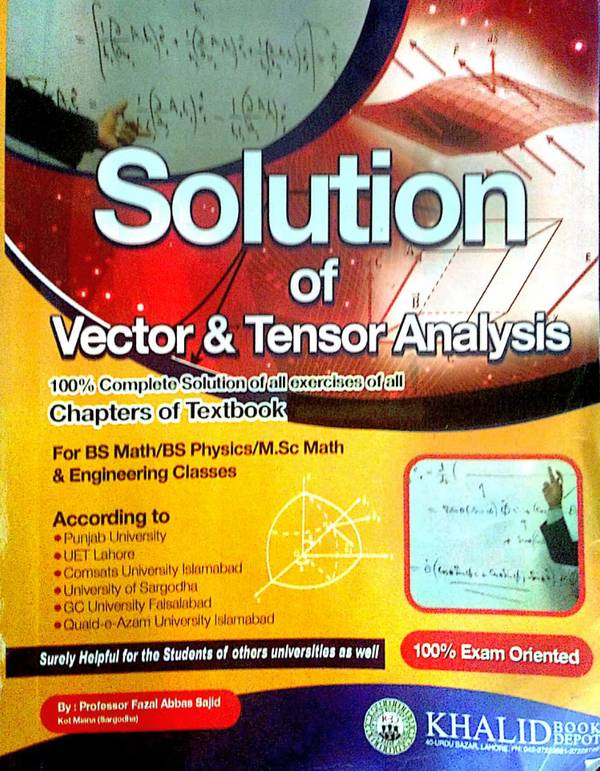# Vector & Tensor Analysis by Prof Fazal AbbasSolution of Chapter 6: Curvilinear Coordinates of the book Vector & Tensor Analysis by Prof. Dr. Nawazish Ali Shah written by Prof. Fazal Abbas Sajid. Here solutions of chapter 6 are provided by the author of the book, for the solutions of all the chapters of the book, please buy the solution manual from the market.

 Name Vector & Tensor Analysis (Chapter 6 only) Prof. Fazal Abbas Sajid 71 pages PDF (see Software section for PDF Reader) 6.17 MB
• Curvilinear coordinates
• Transformation of coordinates
• Coordinate surfaces and coordinate curves
• Unit vectors in curvilinear coordinate system
• Rectangular Cartesian coordinates
• Expressions for arc length, area and volume elements in rectangular Cartesian coordinates
• Expression for Jacobian in rectangular Cartesian coordinates
• Expression for gradient, divergence, curl and Laplacian in rectangular Cartesian coordinates
• Cylindrical polar coordinate
• Cylindrical coordinates in terms of Cartesian coordinates
• Unit vectors in cylindrical coordinate system
• Orthogonally of cylindrical coordinate system
• Relationship among unit vector in cylindrical system

• notes/vector-and-tensor-analysis-by-prof-fazal-abbas